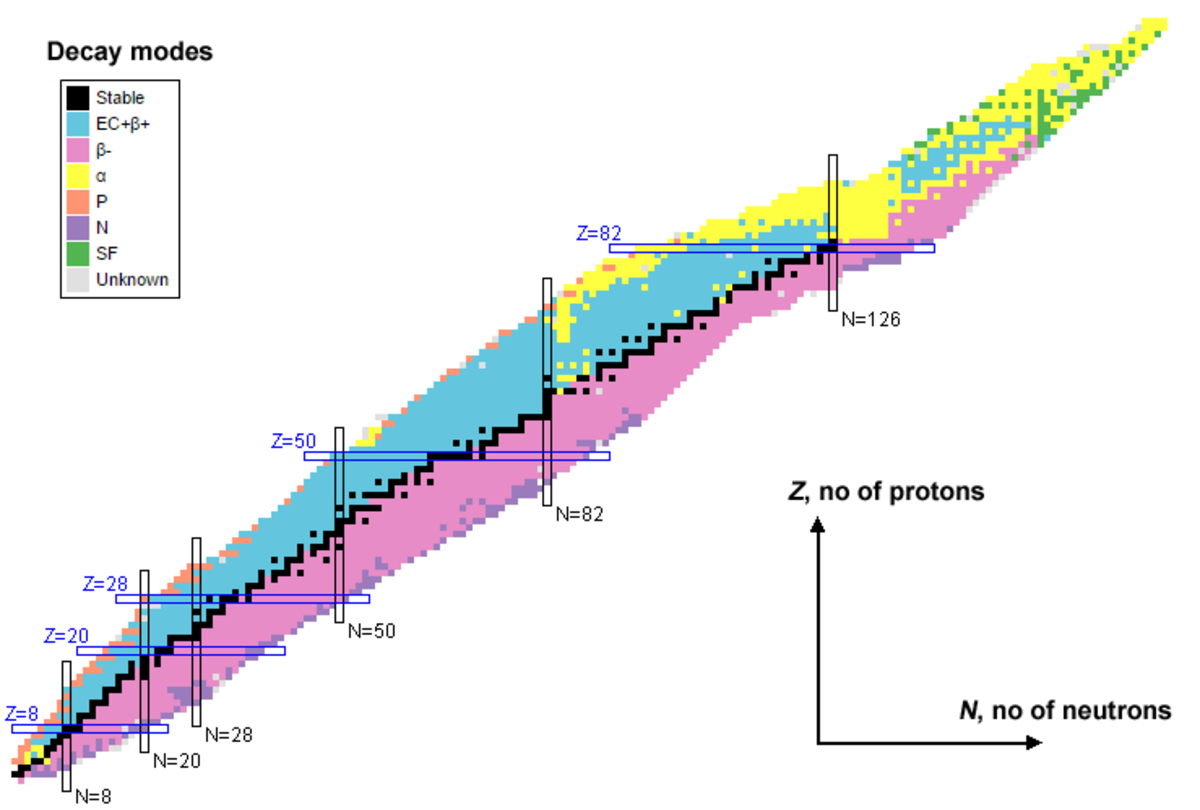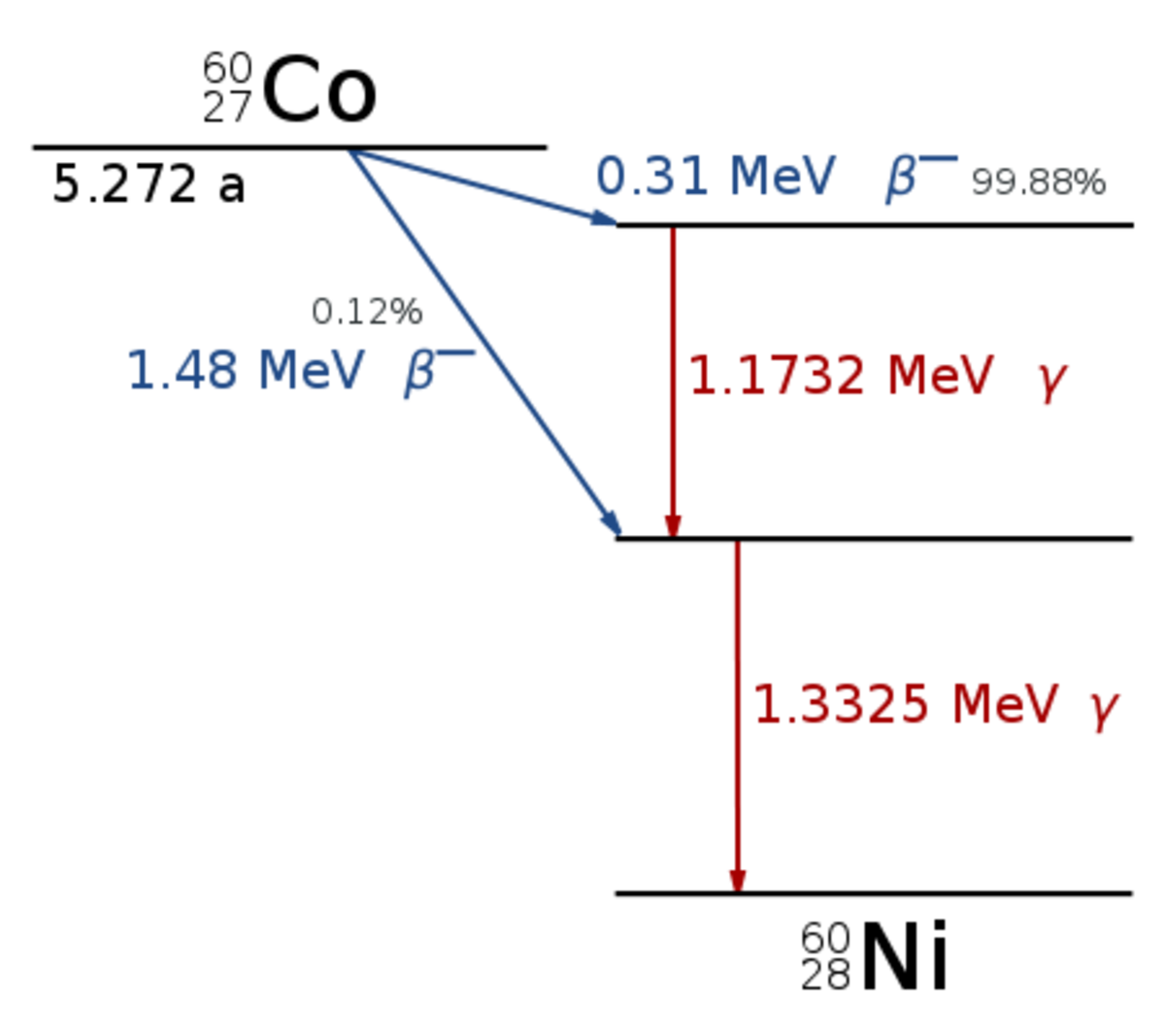Sam Brind holds a master's in physics with theoretical physics (MPhys) from the University of Manchester.

Radioactive materials contain nuclei that are unstable. An unstable nucleus doesn't contain enough binding energy to hold the nucleus together permanently; the cause mostly being the numerical balance of protons and neutrons within the nucleus. The unstable nuclei will randomly undergo processes which lead towards more stable nuclei; these processes are what we call nuclear decay, radioactive decay or just radioactivity.

There are multiple types of decay processes: alpha decay, beta decay, gamma ray emission and nuclear fission. Nuclear fission is the key to nuclear power and atomic bombs. The other three processes lead to the emission of nuclear radiation, which is categorised into three types: alpha particles, beta particles, and gamma rays. All of these types are examples of ionising radiation, radiation with sufficient energy to remove electrons from atoms (creating ions).The table of nuclides (also known as a Segre chart). The key shows the atomic decay modes. The most important are stable atoms (black), alpha decay (yellow), beta minus decay (pink) and electron capture or beta plus decay (blue).National Nuclear Data Centre

## Alpha Particles

An alpha particle consists of two protons and two neutrons bound together (identical to a helium nucleus). Typically, the heaviest nuclides will exhibit alpha decay. The general formula for an alpha decay is shown below.An unstable element, X, decays into a new element, Y, via alpha decay. Note that the new element has two fewer protons and four fewer nucleons.

Alpha particles are the most ionising form of radiation because of their large mass and double charge. Due to this ionising power, they are the most damaging type of radiation to biological tissue. However, this is balanced by alpha particles being the least penetrating type of radiation. Indeed, they will only travel 3-5 cm in air and can be easily stopped by a sheet of paper or your outer layer of dead skin cells. The only way alpha particles can cause serious damage to an organism is by ingestion.

## Beta Particles

A beta particle is simply a high energy electron produced in a beta decay. Unstable nuclei which contain more neutrons than protons (dubbed neutron-rich) can decay via a beta minus decay. The general formula for a beta minus decay is shown below.An unstable element, X, decays into a new element, Y, via beta minus decay. Note that the new element has an additional proton but the number of nucleons (atomic mass) is unchanged. The electron is what we label as a beta minus particle.

Unstable nuclei which are proton-rich can decay towards stability by beta plus decay or electron capture. The beta plus decay results in the emission of an anti-electron (called a positron) which is also classed as a beta particle. The general formulae for both processes are shown below.An unstable element, X, decays into a new element, Y, via beta plus decay. Note that the new element has lost a proton but the number of nucleons (atomic mass) is unchanged. The positron is we label as a beta plus particle.The nucleus of an unstable element, X, captures an inner shell electron to form a new element, Y. Note that the new element has lost a proton but the number of nucleons (atomic mass) is unchanged. No beta particles are emitted in this process.

The properties of beta particles are in the middle of the extremes of alpha particles and gamma rays. They are less ionising than alpha particles but more ionising than gamma rays. Their penetrating power is more than alpha particles but less than gamma rays. Beta particles will travel approximately 15 cm in air and can be stopped by a few mm of aluminium or other materials such as plastic or wood. Care needs to be taken when shielding beta particles with dense materials, as the rapid deceleration of the beta particles will produce gamma rays.

Scroll to Continue

## Gamma Rays

Gamma rays are high energy electromagnetic waves that are emitted when a nucleus decays from an excited state to a lower energy state. The high energy of gamma rays means that they have a very short wavelength and conversely a very high frequency; typically gamma rays have an energy of the order of MeV, which translates to wavelengths of the order of 10-12 m and frequencies of the order of 1020 Hz. Gamma ray emission will normally occur following other nuclear reactions, such as the two previously mentioned decays.The decay scheme for cobalt-60. The cobalt decays through beta decay followed by gamma ray emission to reach the stable state of nickel-60. Other elements have much more complex decay chains.Wikimedia commons

Gamma rays are the least ionising type of radiation, but they are the most penetrating. Theoretically, gamma rays have an infinite range, but the intensity of the rays decrease exponentially with distance, with the rate dependent on the material. Lead is the most effective shielding material, and a few feet will effectively stop the gamma rays. Other materials such as water and dirt can be used but will need to be built up to a larger thickness.

## Biological Effects

Ionising radiation can cause damage to biological tissues. The radiation can directly kill cells, create reactive free radical molecules, damage the DNA and cause mutations such as cancer. The effects of radiation are limited by controlling the dose that people are exposed to. There are three different types of doses that are used depending on the purpose:

• Absorbed dose is the amount of radiation energy deposited in a mass, D = ε/m.Absorbed dose is given in units of grays (1 Gy = 1J/kg).
• Equivalent dose takes into account the biological effects of the radiation by including a radiation weighting factor, ωR, H = ωRD.
• Effective dose also takes into account the type of biological tissue exposed to the radiation by including a tissue weighting factor, ωT, E = ωTωRD. Equivalent and effective doses are given in units of sieverts (1 Sv = 1J/kg).

The dose rate should also be taken into account when determining a radiation risk.

The radiation weighting factors used in calculating an equivalent dose.

gamma rays, beta particles

1

protons

2

heavy ions (such as alpha particles or fission fragments)

20

Some of the tissue weighting factors used in calculating an effective dose. The sum of all the weighting factors be equal to one, for a whole body dose.

Tissue typeTissue weighting factor

stomach, lung, colon, bone marrow

0.12

0.05

skin, bone surface

0.01

The most severe effects of radiation for various whole body doses. The typical level of background radiation is under 10 mSv per year, however the background can reach 100 mSv in some areas of the world.

Radiation dose (single whole body dose)Effect

1 Sv

Temporary depression of blood count.

2 Sv

5 Sv

Death likely within weeks due to bone marrow failure.

10 Sv

Death likely within days due to gastrointestinal damage and infection.

20 Sv

Death likely within hours due to severe nervous system damage.